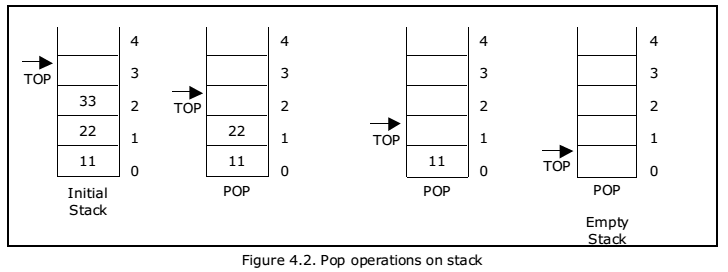# Data Structure :Stack Overview A+ A  A-

Data structure Stack:There are certain situations in computer science that one wants to restrict insertions and deletions so that they can take place only at the beginning or the end of the list, not in the middle. Two of such data structures that are useful are:

• Stack.
• Queue.

Linear lists and arrays allow one to insert and delete elements at any place in the list i.e., at the beginning, at the end or in the middle.

STACK:

A stack is a list of elements in which an element may be inserted or deleted only at one end, called the top of the stack. Stacks are sometimes known as LIFO (last in, first out) lists.

As the items can be added or removed only from the top i.e. the last item to be added to a stack is the first item to be removed.

The two basic operations associated with stacks are:

• Push: is the term used to insert an element into a stack.
• Pop: is the term used to delete an element from a stack.

Push” is the term used to insert an element into a stack. “Pop” is the term used to delete an element from the stack.

All insertions and deletions take place at the same end, so the last element added to the stack will be the first element removed from the stack. When a stack is created, the stack base remains fixed while the stack top changes as elements are added and removed. The most accessible element is the top and the least accessible element is the bottom of the stack.

Representation of Stack:

Let us consider a stack with 6 elements capacity. This is called as the size of the stack. The number of elements to be added should not exceed the maximum size of the stack. If we attempt to add new element beyond the maximum size, we will encounter a stack overflow condition. Similarly, you cannot remove elements beyond the base of the stack. If such is the case, we will reach a stack underflow condition.

When an element is added to a stack, the operation is performed by push(). Figure 4.1 shows the creation of a stack and addition of elements using push().When an element is taken off from the stack, the operation is performed by pop(). Figure 4.2 shows a stack initially with three elements and shows the deletion of elements using pop().We can represent a stack as a linked list. In a stack push and pop operations are performed at one end called top. We can perform similar operations at one end of list using top pointer. The linked stack looks as shown in figure 4.3.Applications of stacks:
1. Stack is used by compilers to check for balancing of parentheses, brackets
and braces.
2. Stack is used to evaluate a postfix expression.
3. Stack is used to convert an infix expression into postfix/prefix form.
4. In recursion, all intermediate arguments and return values are stored on the
processor’s stack.
5. During a function call the return address and arguments are pushed onto a
stack and on return they are popped off.